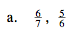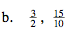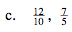### Home > MC2 > Chapter 7 > Lesson 7.1.6 > Problem7-57

7-57.
1. For each pair of slope ratios, decide if they are equivalent (=), or if one slope is greater. If the slopes are not equal, use the greater than (>) or less than (<) symbol to show which is greater. Homework Help ✎

1.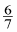,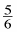2.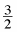,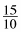3.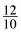,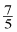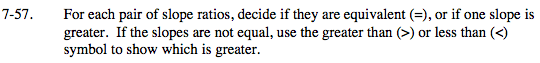Find a common denominator
for the fractions.
Let's use 42.

$\text{Multiply \frac{6}{7}\: by\: \frac{6}{6}\: and\: \frac{5}{6}\: by\: \frac{7}{7}.}$

Compare to find the greatest fraction.